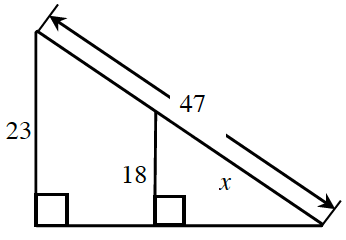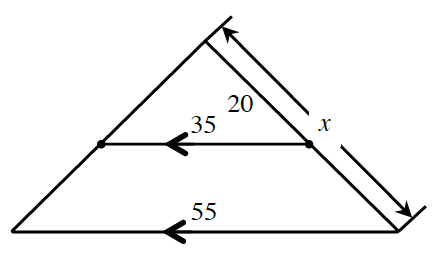### Home > INT3 > Chapter 4 > Lesson 4.2.1 > Problem4-49

4-49.

Solve for $x$ in the following diagrams.

1.The triangles are similar. Recall that in similar triangles corresponding sides are proportional.

$\frac{18}{x} = \frac{23}{47}$

1.See part (a). Try drawing two separate triangles.

$x \approx 31.43$